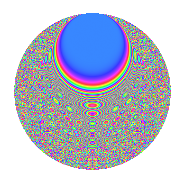# Properties

 Label 8011.2.aLevel 8011 Weight 2 Character orbit a Rep. character $$\chi_{8011}(1,\cdot)$$ Character field $$\Q$$ Dimension 667 Newforms 2 Sturm bound 1335 Trace bound 1

# Related objects

## Defining parameters

 Level: $$N$$ = $$8011$$ Weight: $$k$$ = $$2$$ Character orbit: $$[\chi]$$ = 8011.a (trivial) Character field: $$\Q$$ Newforms: $$2$$ Sturm bound: $$1335$$ Trace bound: $$1$$

## Dimensions

The following table gives the dimensions of various subspaces of $$M_{2}(\Gamma_0(8011))$$.

Total New Old
Modular forms 668 668 0
Cusp forms 667 667 0
Eisenstein series 1 1 0

The following table gives the dimensions of the cuspidal new subspaces with specified eigenvalues for the Atkin-Lehner operators.

$$8011$$Dim.
$$+$$$$309$$
$$-$$$$358$$

## Trace form

 $$667q - 4q^{3} + 664q^{4} + 2q^{5} + 6q^{8} + 665q^{9} + O(q^{10})$$ $$667q - 4q^{3} + 664q^{4} + 2q^{5} + 6q^{8} + 665q^{9} - 2q^{10} - 2q^{11} - 22q^{12} - 4q^{13} - 8q^{14} - 16q^{15} + 654q^{16} + 2q^{17} + 4q^{18} - 14q^{19} + 2q^{20} + 2q^{21} - 14q^{22} + 10q^{23} + 669q^{25} + 8q^{26} - 16q^{27} + 10q^{28} + 28q^{29} + 14q^{30} - 6q^{31} + 22q^{32} - 2q^{34} - 4q^{35} + 664q^{36} + 2q^{37} - 2q^{39} - 12q^{40} + 6q^{41} - 16q^{42} - 18q^{43} - 10q^{44} + 6q^{45} + 10q^{46} - 16q^{47} - 76q^{48} + 665q^{49} - 22q^{50} - 22q^{51} - 22q^{52} - 2q^{53} - 50q^{54} - 20q^{55} - 42q^{56} - 40q^{57} + 44q^{58} - 24q^{59} - 62q^{60} - 10q^{61} - 38q^{62} - 30q^{63} + 650q^{64} + 8q^{65} - 80q^{66} - 24q^{67} - 10q^{68} + 6q^{69} + 12q^{70} - 8q^{71} + 10q^{72} - 12q^{73} + 36q^{74} - 38q^{75} - 58q^{76} + 60q^{77} - 8q^{78} + 2q^{79} - 8q^{80} + 675q^{81} + 50q^{82} + 4q^{83} + 22q^{84} + 6q^{85} + 6q^{86} + 22q^{87} + 14q^{89} + 56q^{90} - 52q^{91} + 46q^{92} - 36q^{94} + 4q^{95} + 44q^{96} + 10q^{97} + 80q^{98} - 2q^{99} + O(q^{100})$$

## Decomposition of $$S_{2}^{\mathrm{new}}(\Gamma_0(8011))$$ into irreducible Hecke orbits

Label Dim. $$A$$ Field CM Traces A-L signs $q$-expansion
$$a_2$$ $$a_3$$ $$a_5$$ $$a_7$$ 8011
8011.2.a.a $$309$$ $$63.968$$ None $$-33$$ $$-15$$ $$-74$$ $$-19$$ $$+$$
8011.2.a.b $$358$$ $$63.968$$ None $$33$$ $$11$$ $$76$$ $$19$$ $$-$$